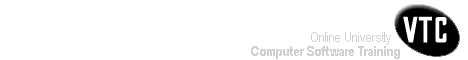Help Centre/Articles: Home & Garden Arts & Crafts
Engineering Resources:
Project ManagementPrevious Page 3 of 3 Chapter 09 | 1 of 3 Chapter 10 | Next Page 2 of 3 Chapter 10Home > Resources > Project Management for Construction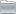Project ManagementProject Management 01) The Owners' Perspective 02) Organizing For Project Management Page 02 of 02 Chapter 02 03) The Design And Construction Process 04) Labor, Material, And Equipment Utilization 05) Cost Estimation 06) Economic Evaluation of Facility Investments 07) Financing of Constructed Facilities 08) Construction Pricing and Contracting 09) Construction Planning 10) Fundamental Scheduling Procedures 11) Advanced Scheduling Techniques 12) Cost Control, Monitoring, and Accounting 13) Quality Control and Safety During Construction 14) Organization and Use of Project Information10. Fundamental Scheduling Procedures-01

# 10. Fundamental Scheduling Procedures

## 10.1 Relevance of Construction Schedules

In addition to assigning dates to project activities, project scheduling is intended to match the resources of equipment, materials and labor with project work tasks over time. Good scheduling can eliminate problems due to production bottlenecks, facilitate the timely procurement of necessary materials, and otherwise insure the completion of a project as soon as possible. In contrast, poor scheduling can result in considerable waste as laborers and equipment wait for the availability of needed resources or the completion of preceding tasks. Delays in the completion of an entire project due to poor scheduling can also create havoc for owners who are eager to start using the constructed facilities.

Attitudes toward the formal scheduling of projects are often extreme. Many owners require detailed construction schedules to be submitted by contractors as a means of monitoring the work progress. The actual work performed is commonly compared to the schedule to determine if construction is proceeding satisfactorily. After the completion of construction, similar comparisons between the planned schedule and the actual accomplishments may be performed to allocate the liability for project delays due to changes requested by the owner, worker strikes or other unforeseen circumstances.

In contrast to these instances of reliance upon formal schedules, many field supervisors disdain and dislike formal scheduling procedures. In particular, the critical path method of scheduling is commonly required by owners and has been taught in universities for over two decades, but is often regarded in the field as irrelevant to actual operations and a time consuming distraction. The result is "seat-of-the-pants" scheduling that can be good or that can result in grossly inefficient schedules and poor productivity. Progressive construction firms use formal scheduling procedures whenever the complexity of work tasks is high and the coordination of different workers is required.

Formal scheduling procedures have become much more common with the advent of personal computers on construction sites and easy-to-use software programs. Sharing schedule information via the Internet has also provided a greater incentive to use formal scheduling methods. Savvy construction supervisors often carry schedule and budget information around with wearable or handheld computers. As a result, the continued development of easy to use computer programs and improved methods of presenting schedules hav overcome the practical problems associated with formal scheduling mechanisms. But problems with the use of scheduling techniques will continue until managers understand their proper use and limitations.

A basic distinction exists between resource oriented and time oriented scheduling techniques. For resource oriented scheduling, the focus is on using and scheduling particular resources in an effective fashion. For example, the project manager's main concern on a high-rise building site might be to insure that cranes are used effectively for moving materials; without effective scheduling in this case, delivery trucks might queue on the ground and workers wait for deliveries on upper floors. For time oriented scheduling, the emphasis is on determining the completion time of the project given the necessary precedence relationships among activities. Hybrid techniques for resource leveling or resource constrained scheduling in the presence of precedence relationships also exist. Most scheduling software is time-oriented, although virtually all of the programs have the capability to introduce resource constaints.

This chapter will introduce the fundamentals of scheduling methods. Our discussion will generally assume that computer based scheduling programs will be applied. Consequently, the wide variety of manual or mechanical scheduling techniques will not be discussed in any detail. These manual methods are not as capable or as convenient as computer based scheduling. With the availability of these computer based scheduling programs, it is important for managers to understand the basic operations performed by scheduling programs. Moreover, even if formal methods are not applied in particular cases, the conceptual framework of formal scheduling methods provides a valuable reference for a manager. Accordingly, examples involving hand calculations will be provided throughout the chapter to facilitate understanding.

## 10.2 The Critical Path Method

The most widely used scheduling technique is the critical path method (CPM) for scheduling, often referred to as critical path scheduling. This method calculates the minimum completion time for a project along with the possible start and finish times for the project activities. Indeed, many texts and managers regard critical path scheduling as the only usable and practical scheduling procedure. Computer programs and algorithms for critical path scheduling are widely available and can efficiently handle projects with thousands of activities.

The critical path itself represents the set or sequence of predecessor/successor activities which will take the longest time to complete. The duration of the critical path is the sum of the activities' durations along the path. Thus, the critical path can be defined as the longest possible path through the "network" of project activities, as described in Chapter 9. The duration of the critical path represents the minimum time required to complete a project. Any delays along the critical path would imply that additional time would be required to complete the project.

There may be more than one critical path among all the project activities, so completion of the entire project could be delayed by delaying activities along any one of the critical paths. For example, a project consisting of two activities performed in parallel that each require three days would have each activity critical for a completion in three days.

Formally, critical path scheduling assumes that a project has been divided into activities of fixed duration and well defined predecessor relationships. A predecessor relationship implies that one activity must come before another in the schedule. No resource constraints other than those implied by precedence relationships are recognized in the simplest form of critical path scheduling.

To use critical path scheduling in practice, construction planners often represent a resource constraint by a precedence relation. A constraint is simply a restriction on the options available to a manager, and a resource constraint is a constraint deriving from the limited availability of some resource of equipment, material, space or labor. For example, one of two activities requiring the same piece of equipment might be arbitrarily assumed to precede the other activity. This artificial precedence constraint insures that the two activities requiring the same resource will not be scheduled at the same time. Also, most critical path scheduling algorithms impose restrictions on the generality of the activity relationships or network geometries which are used. In essence, these restrictions imply that the construction plan can be represented by a network plan in which activities appear as nodes in a network, as in Figure 9-6. Nodes are numbered, and no two nodes can have the same number or designation. Two nodes are introduced to represent the start and completion of the project itself.

The actual computer representation of the project schedule generally consists of a list of activities along with their associated durations, required resources and predecessor activities. Graphical network representations rather than a list are helpful for visualization of the plan and to insure that mathematical requirements are met. The actual input of the data to a computer program may be accomplished by filling in blanks on a screen menu, reading an existing datafile, or typing data directly to the program with identifiers for the type of information being provided.

With an activity-on-branch network, dummy activities may be introduced for the purposes of providing unique activity designations and maintaining the correct sequence of activities. A dummy activity is assumed to have no time duration and can be graphically represented by a dashed line in a network. Several cases in which dummy activities are useful are illustrated in Fig. 10-1. In Fig. 10-1(a), the elimination of activity C would mean that both activities B and D would be identified as being between nodes 1 and 3. However, if a dummy activity X is introduced, as shown in part (b) of the figure, the unique designations for activity B (node 1 to 2) and D (node 1 to 3) will be preserved. Furthermore, if the problem in part (a) is changed so that activity E cannot start until both C and D are completed but that F can start after D alone is completed, the order in the new sequence can be indicated by the addition of a dummy activity Y, as shown in part (c). In general, dummy activities may be necessary to meet the requirements of specific computer scheduling algorithms, but it is important to limit the number of such dummy link insertions to the extent possible.

##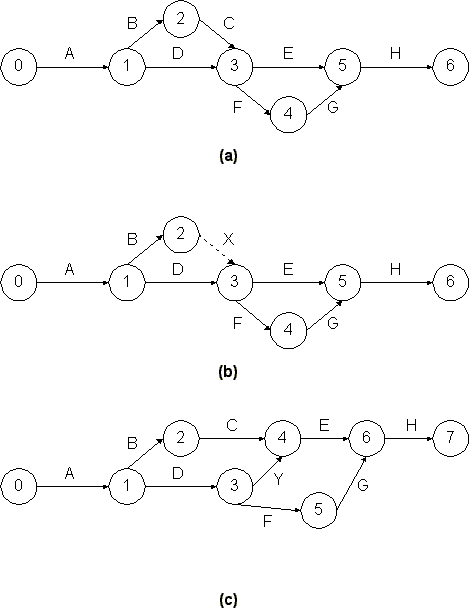Figure 10-1  Dummy Activities in a Project Network

Many computer scheduling systems support only one network representation, either activity-on-branch or acitivity-on-node. A good project manager is familiar with either representation.

Example 10-1: Formulating a network diagram

Suppose that we wish to form an activity network for a seven-activity network with the following precedences:

 Activity Predecessors A B C D E F G --- --- A,B C C D D,E

Forming an activity-on-branch network for this set of activities might begin be drawing activities A, B and C as shown in Figure 10-2(a). At this point, we note that two activities (A and B) lie between the same two event nodes; for clarity, we insert a dummy activity X and continue to place other activities as in Figure 10-2(b). Placing activity G in the figure presents a problem, however, since we wish both activity D and activity E to be predecessors. Inserting an additional dummy activity Y along with activity G completes the activity network, as shown in Figure 10-2(c). A comparable activity-on-node representation is shown in Figure 10-3, including project start and finish nodes. Note that dummy activities are not required for expressing precedence relationships in activity-on-node networks.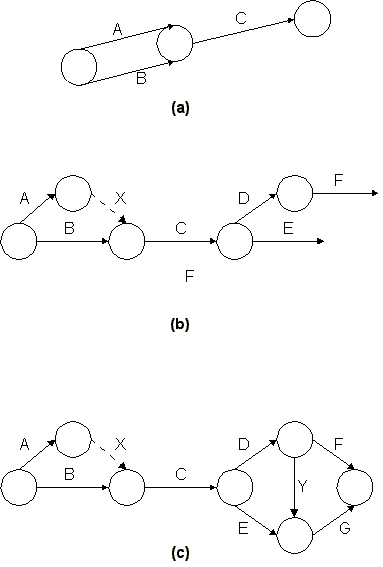Figure 10-2  An Activity-on-Branch Network for Critical Path Scheduling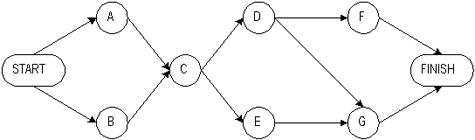Figure 10-3  An Activity-on-Node Network for Critical Path Scheduling

## 10.3 Calculations for Critical Path Scheduling

With the background provided by the previous sections, we can formulate the critical path scheduling mathematically. We shall present an algorithm or set of instructions for critical path scheduling assuming an activity-on-branch project network. We also assume that all precedences are of a finish-to-start nature, so that a succeeding activity cannot start until the completion of a preceding activity. In a later section, we present a comparable algorithm for activity-on-node representations with multiple precedence types.

Suppose that our project network has n+1 nodes, the initial event being 0 and the last event being n. Let the time at which node events occur be x1, x2,...., xn, respectively. The start of the project at x0 will be defined as time 0. Nodal event times must be consistent with activity durations, so that an activity's successor node event time must be larger than an activity's predecessor node event time plus its duration. For an activity defined as starting from event i and ending at event j, this relationship can be expressed as the inequality constraint, xj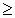xi + Dij where Dij is the duration of activity (i,j). This same expression can be written for every activity and must hold true in any feasible schedule. Mathematically, then, the critical path scheduling problem is to minimize the time of project completion (xn) subject to the constraints that each node completion event cannot occur until each of the predecessor activities have been completed:

Minimize

(10.1)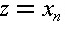subject toThis is a linear programming problem since the objective value to be minimized and each of the constraints is a linear equation.

Rather than solving the critical path scheduling problem with a linear programming algorithm (such as the Simplex method), more efficient techniques are available that take advantage of the network structure of the problem. These solution methods are very efficient with respect to the required computations, so that very large networks can be treated even with personal computers. These methods also give some very useful information about possible activity schedules. The programs can compute the earliest and latest possible starting times for each activity which are consistent with completing the project in the shortest possible time. This calculation is of particular interest for activities which are not on the critical path (or paths), since these activities might be slightly delayed or re-scheduled over time as a manager desires without delaying the entire project.

An efficient solution process for critical path scheduling based upon node labeling is shown in Table 10-1. Three algorithms appear in the table. The event numbering algorithm numbers the nodes (or events) of the project such that the beginning event has a lower number than the ending event for each activity. Technically, this algorithm accomplishes a "topological sort" of the activities. The project start node is given number 0. As long as the project activities fulfill the conditions for an activity-on-branch network, this type of numbering system is always possible. Some software packages for critical path scheduling do not have this numbering algorithm programmed, so that the construction project planners must insure that appropriate numbering is done.

TABLE 10-1  Critical Path Scheduling Algorithms (Activity-on-Branch Representation)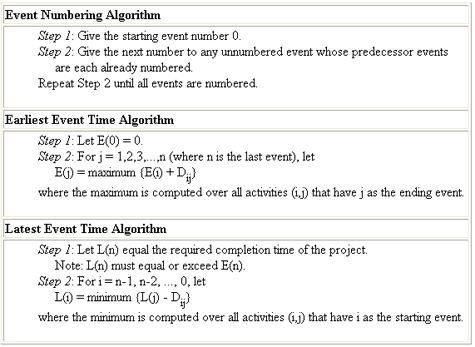The earliest event time algorithm computes the earliest possible time, E(i), at which each event, i, in the network can occur. Earliest event times are computed as the maximum of the earliest start times plus activity durations for each of the activities immediately preceding an event. The earliest start time for each activity (i,j) is equal to the earliest possible time for the preceding event E(i):

(10.2)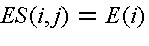The earliest finish time of each activity (i,j) can be calculated by:

(10.3)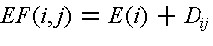Activities are identified in this algorithm by the predecessor node (or event) i and the successor node j. The algorithm simply requires that each event in the network should be examined in turn beginning with the project start (node 0).

The latest event time algorithm computes the latest possible time, L(j), at which each event j in the network can occur, given the desired completion time of the project, L(n) for the last event n. Usually, the desired completion time will be equal to the earliest possible completion time, so that E(n) = L(n) for the final node n. The procedure for finding the latest event time is analogous to that for the earliest event time except that the procedure begins with the final event and works backwards through the project activities. Thus, the earliest event time algorithm is often called a forward pass through the network, whereas the latest event time algorithm is the the backward pass through the network. The latest finish time consistent with completion of the project in the desired time frame of L(n) for each activity (i,j) is equal to the latest possible time L(j) for the succeeding event:

(10.4)The latest start time of each activity (i,j) can be calculated by:

(10.5)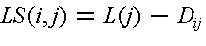The earliest start and latest finish times for each event are useful pieces of information in developing a project schedule. Events which have equal earliest and latest times, E(i) = L(i), lie on the critical path or paths. An activity (i,j) is a critical activity if it satisfies all of the following conditions:

(10.6)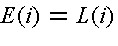(10.7)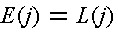(10.8)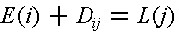Hence, activities between critical events are also on a critical path as long as the activity's earliest start time equals its latest start time, ES(i,j) = LS(i,j). To avoid delaying the project, all the activities on a critical path should begin as soon as possible, so each critical activity (i,j) must be scheduled to begin at the earliest possible start time, E(i).

Example 10-2: Critical path scheduling calculations

Consider the network shown in Figure 10-4 in which the project start is given number 0. Then, the only event that has each predecessor numbered is the successor to activity A, so it receives number 1. After this, the only event that has each predecessor numbered is the successor to the two activities B and C, so it receives number 2. The other event numbers resulting from the algorithm are also shown in the figure. For this simple project network, each stage in the numbering process found only one possible event to number at any time. With more than one feasible event to number, the choice of which to number next is arbitrary. For example, if activity C did not exist in the project for Figure 10-4, the successor event for activity A or for activity B could have been numbered 1.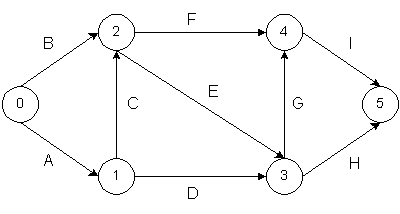Figure 10-4  A Nine-Activity Project Network

Once the node numbers are established, a good aid for manual scheduling is to draw a small rectangle near each node with two possible entries. The left hand side would contain the earliest time the event could occur, whereas the right hand side would contain the latest time the event could occur without delaying the entire project. Figure 10-5 illustrates a typical box.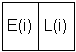Figure 10-5  E(i) and L(i) Display for Hand Calculation of Critical Path for Activity-on-Branch Representation

TABLE 10-2  Precedence Relations and Durations for a Nine Activity Project ExampleFor the network in Figure 10-4 with activity durations in Table 10-2, the earliest event time calculations proceed as follows:

 Step1E(0) = 0 Step 2 j = 1E(1) = Max{E(0) + D01} = Max{ 0 + 4 } = 4 j = 2E(2) = Max{E(0) + D02; E(1) + D12} = Max{0 + 3; 4 + 8} = 12 j = 3E(3) = Max{E(1) + D13; E(2) + D23} = Max{4 + 7; 12 + 9} = 21 j = 4E(4) = Max{E(2) + D24; E(3) + D34} = Max{12 + 12; 21 + 2} = 24 j = 5E(5) = Max{E(3) + D35; E(4) + D45} = Max{21 + 5; 24 + 6} = 30

Thus, the minimum time required to complete the project is 30 since E(5) = 30. In this case, each event had at most two predecessors.

For the "backward pass," the latest event time calculations are:

 Step 1L(5) = E(5) = 30 Step 2 j = 4L(4) = Min {L(5) - D45} = Min {30 - 6} = 24 j = 3L(3) = Min {L(5) - D35; L(4) - D34} = Min {30 -5; 24 - 2} = 22 j = 2L(2) = Min {L(4) - D24; L(3) - D23} = Min {24 - 12; 22 - 9} = 12 j = 1L(1) = Min {L(3) - D13; L(2) - D12} = Min {22 - 7; 12 - 8} = 4 j = 0L(0) = Min {L(2) - D02; L(1) - D01} = Min {12 - 3; 4 - 4} = 0

In this example, E(0) = L(0), E(1) = L(1), E(2) = L(2), E(4) = L(4),and E(5) = L(5). As a result, all nodes but node 3 are in the critical path. Activities on the critical path include A (0,1), C (1,2), F (2,4) and I (4,5) as shown in Table 10-3.

TABLE 10-3  Identification of Activities on the Critical Path for a Nine-Activity Project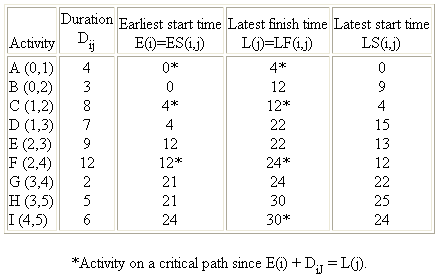Previous Page 3 of 3 Chapter 09 | 1 of 3 Chapter 10 | Next Page 2 of 3 Chapter 10Sketch-Plus Home  |  Contacts  |  Samples  |  Products  | Books  |  SitemapSketch-Plus.com © 2004 | Privacy Policy | Terms of Use
Fundamental Scheduling Procedures-01